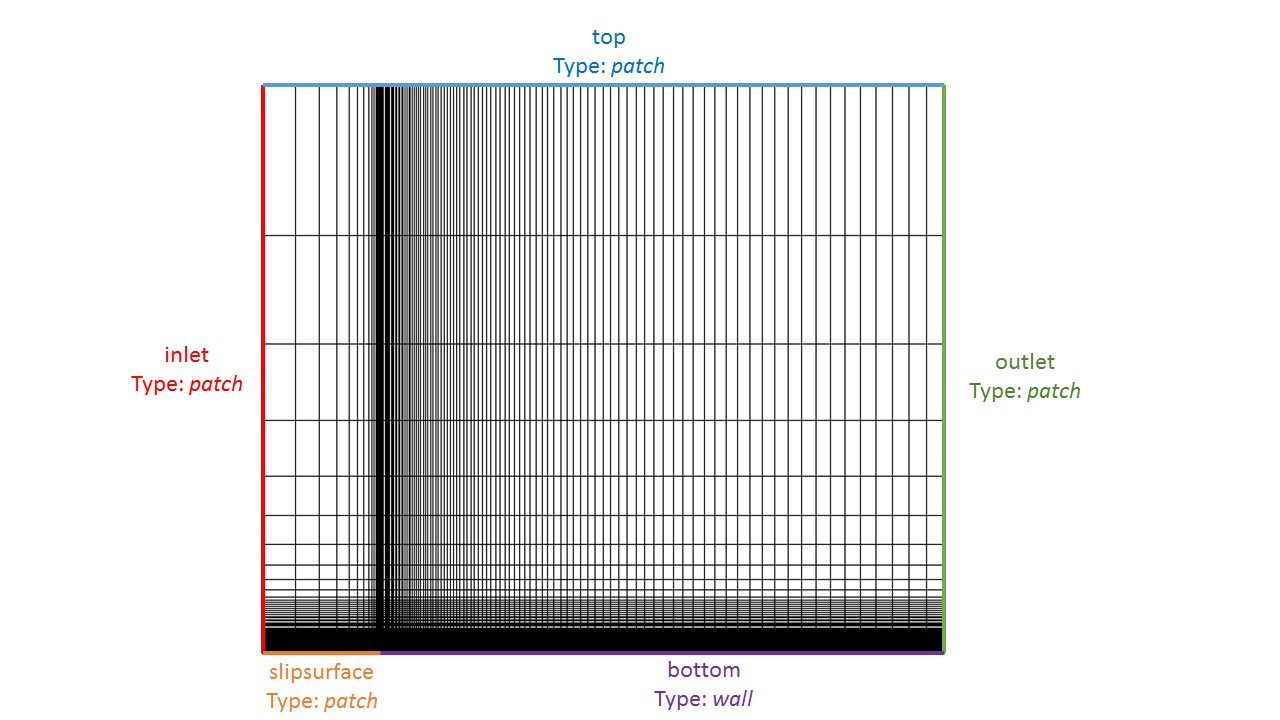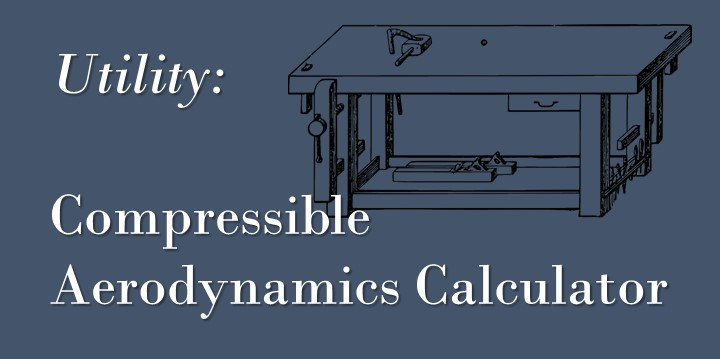Skip to content# CategoryUncategorized

## Equations for Steady 1D Isentropic Flow

The equations used to describe steady 1D isentropic flow are derived from conservation of mass, momentum, and energy, as well as an equation of state (typically the ideal gas law). … Continue Reading Equations for Steady 1D Isentropic Flow## Turbulent Zero Pressure Gradient Flat Plate – simpleFOAM, komegaSST

An excellent test case, and case to familiarize yourself with some of the turbulence models available in OpenFOAM is a 2D flat plate with zero pressure gradient. I will solve … Continue Reading Turbulent Zero Pressure Gradient Flat Plate – simpleFOAM, komegaSST## Compressible Aerodynamics Calculator – Matlab

Here I have written a Matlab App for calculating compressible aerodynamics using the basic gas dynamics equations. The calculations available are: Isentropic Flow (including Prandtl-Meyer flow) Normal Shocks (See article) … Continue Reading Compressible Aerodynamics Calculator – Matlab